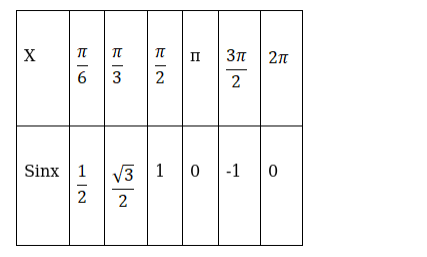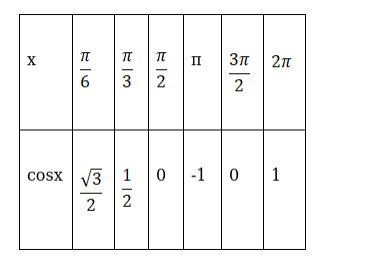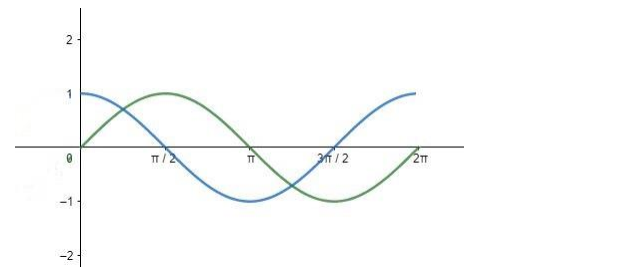# Draw the graphs of y = sin x andQuestion:

Draw the graphs of y = sin x and $y=\cos x$ in $[0,2 \pi]$ on the same axes.

Solution:

For sinxFor cosxThe green line represents curve for $\sin (x)$ and blue for $\cos (x)$ for $[0,2 \pi]$.